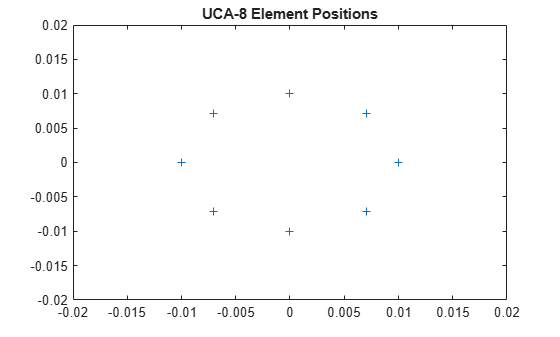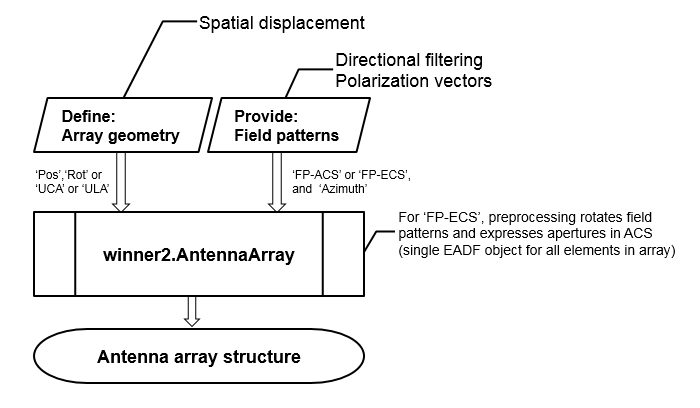# winner2.AntennaArray

Create antenna array for WINNER II channel model

## Syntax

``antArray = winner2.AntennaArray``
``antArray = winner2.AntennaArray(Name,Value)``

## Description

````antArray = winner2.AntennaArray` returns a structure representing an antenna array with one isotropic antenna element. Both the antenna array and the single element have no rotation and are located at the origin, [0;0;0]. ```

example

````antArray = winner2.AntennaArray(Name,Value)` returns a structure representing an antenna array defined using one or more `Name,Value` pair arguments.For more information, see Antenna Array Model.```

## Examples

collapse all

Use the `winner2.AntennaArray` function to create an eight element uniform circular array (UCA-8) with a 1 cm radius.

`UCA8 = winner2.AntennaArray('UCA',8,0.01);`

Plot element positions.

```pos = {UCA8.Element(:).Pos}; plot(cellfun(@(x) x(1),pos),cellfun(@(x) x(2),pos),'+'); xlim([-0.02 0.02]); ylim([-0.02 0.02]); title('UCA-8 Element Positions');```Use the `winner2.AntennaArray` function to create a two element uniform linear array (ULA-2) with 50 cm spacing and the dipole elements slanted at +45 and -45 degrees.

```az = -180:179; % 1-degree spacing pattern = cat(1,shiftdim(winner2.dipole(az,45),-1), ... shiftdim(winner2.dipole(az,-45),-1)); ULA2 = winner2.AntennaArray('ULA',2,0.5, ... 'FP-ECS',pattern,'Azimuth',az);```

## Input Arguments

collapse all

### Name-Value Arguments

Specify optional pairs of arguments as `Name1=Value1,...,NameN=ValueN`, where `Name` is the argument name and `Value` is the corresponding value. Name-value arguments must appear after other arguments, but the order of the pairs does not matter.

Before R2021a, use commas to separate each name and value, and enclose `Name` in quotes.

Example: `'Pos',[1 0 0; 0 1 0],'Rot',[0 0 0; 0 pi() 0]` indicates the coordinates and rotation angles for two antenna elements.

Position of each antenna element, specified as the comma-separated pair consisting of `'Pos'` and a column vector or an NE-by-3 matrix. The three columns represent the x-, y-, and z-coordinates in meters from the origin. NE indicates the number of elements in the antenna array. The elements have no rotation. When there is more than one element, the `'Element'` field of `antArray` is a row vector of structures representing all the elements.

Example: `'Pos',[63.1 10.2 11.5; 62 11 12]` indicates the coordinates for two antenna elements.

Data Types: `double`

Rotation angle of each antenna element, specified as the comma-separated pair consisting of `'Rot'` and a column vector or an NE-by-3 matrix. The three columns represent the RotX, RotY, and RotZ rotation angles of each antenna element in radians. NE indicates the number of elements in the antenna array. `Rot` only applies when `Pos` is specified. If not specified with `Pos`, the rotation angle is `0`.

Example: `'Rot',[2 1.5 0; 0 pi() 0]` indicates the rotation angles for two antenna elements.

Data Types: `double`

Uniform circular antenna array, specified as the comma-separated pair consisting of `'UCA'` and `N,Rad`. In this argument, `N` indicates the number of elements (NE) and `Rad` indicates the radius in meters. If `Rad` is not specified, the default radius is 1 meter.

Example: `'UCA',8,0.5` indicates an eight element uniform circular array with 0.5 meter radius.

Data Types: `double`

Uniform linear antenna array, specified as the comma-separated pair consisting of `'ULA'` and `N,Spacing`. In this argument, `N` indicates the number of elements (NE) and `Spacing` indicates the separation between adjacent elements in meters. If `Spacing` is not specified, the default separation is 1/`N` meters.

ULA elements are placed along x-axis with the center of the array at [0;0;0]. For an even number of elements, there is no antenna element at [0;0;0].

Example: `'ULA',3,0.25` indicates a three element uniform linear array with 0.25 meter spacing between adjacent elements.

Data Types: `double`

Field pattern of element coordinate system, specified as the comma-separated pair consisting of `'FP-ECS'` and a P-by-2-by1-by-NAZ array.

• The first dimension, P, can be either 1 or any number greater than or equal to the number of elements in the antenna array (NE). When P = 1, the same pattern applies to all elements. When P > NE, the first NE rows apply.

• The second dimension, `2`, indicates that two polarizations characterize the field pattern. The first dimension in the field pattern stores vertical polarization, and the second one stores horizontal polarization.

• The third dimension, `1`, indicates that one elevation angle characterizes the field pattern.

• The fourth dimension, NAZ, is the number of field pattern samples taken between –180 and 180 degrees. NAZ equals the number of elements specified in `Azimuth` or when `Azimuth` is not present it equals the number of equidistant field pattern samples taken over azimuth angle.

Data Types: `double`

Field pattern array coordinate system, specified as the comma-separated pair consisting of `'FP-ACS'` and a P-by-2-by1-by-NAZ array. Array format is the same as the `FP-ECS` syntax, except that the field pattern is specified in the array-coordinate-system (ACS).

• The first dimension, P, can be either 1 or any number greater than or equal to the number of elements in the antenna array (NE). When P = 1, the same pattern applies to all elements. When P > NE, the first NE rows apply.

• The second dimension, `2`, indicates that two polarizations characterize the field pattern. The first dimension in the field pattern stores vertical polarization, and the second one stores horizontal polarization. Missing polarization dimensions of the field pattern are substituted with zeros.

• The third dimension, `1`, indicates that one elevation angle characterizes the field pattern.

• The fourth dimension, NAZ, is the number of field pattern samples taken between –180 and 180 degrees. NAZ equals the number of elements specified in `Azimuth` or when `Azimuth` is not present it equals the number of equidistant field pattern samples taken over azimuth angle.

Data Types: `double`

Azimuth angles for `FP-ACS` or `FP-ECS` field patterns in degrees , specified as the comma-separated pair consisting of `'Azimuth'` and an 1-by-NAZ row vector. The values in the row vector indicate azimuth angles for elements in the field patterns.

Note

`Azimuth` applies only when `FP-ACS` or `FP-ECS` are defined. If `Azimuth` is not specified, uniform spacing is used for elements in the field pattern.

Example: `'Azimuth',[0 10 20 90 180 270 340 350]`

Data Types: `double`

## Output Arguments

collapse all

Antenna array definition, returned as a structure containing these fields.

Antenna array name, returned as a character vector.

Antenna array position, returned as a 3-by-1 vector, representing the x-, y-, and z-coordinates in meters from the origin.

Antenna array rotation, returned as a 3-by-1 vector, representing the RotX, RotY, and RotZ rotation angles of each antenna element in radians.

Element definition, returned as a row vector of structures, with each structure representing one element and containing these fields.

Antenna array position, returned as a 3-by-1 vector, representing the x-, y-, and z-coordinates in meters from the origin.

Antenna array rotation, returned as a 3-by-1 vector, representing the RotX, RotY, and RotZ rotation angles of each antenna element in radians.

Aperture definition, returned as a structure representing the antenna aperture.

To create an antenna array model, you must define the geometry of array elements (positions and rotation) and the element field patterns. The arguments provided to `winner2.AntennaArray` are always processed such that the array geometry is created first, and then the field patterns are assigned.